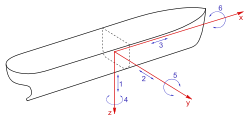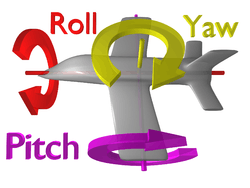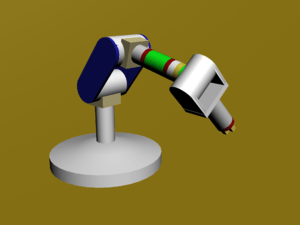# Degrees of freedom (mechanics)

In physics, the degree of freedom (DOF) of a mechanical system is the number of independent parameters that define its configuration. It is the number of parameters that determine the state of a physical system and is important to the analysis of systems of bodies in mechanical engineering, aeronautical engineering, robotics, and structural engineering.

The position of a single car (engine) moving along a track has one degree of freedom because the position of the car is defined by the distance along the track. A train of rigid cars connected by hinges to an engine still has only one degree of freedom because the positions of the cars behind the engine are constrained by the shape of the track.

An automobile with highly stiff suspension can be considered to be a rigid body traveling on a plane (a flat, two-dimensional space). This body has three independent degrees of freedom consisting of two components of translation and one angle of rotation. Skidding or drifting is a good example of an automobile's three independent degrees of freedom.

The position and orientation of a rigid body in space is defined by three components of translation and three components of rotation, which means that it has six degrees of freedom.

The exact constraint mechanical design method manages the degrees of freedom to neither underconstrain nor overconstrain a device.

## Motions and dimensions

The position of an n-dimensional rigid body is defined by the rigid transformation, [T] = [A, d], where d is an n-dimensional translation and A is an n × n rotation matrix, which has n translational degrees of freedom and n(n  1)/2 rotational degrees of freedom. The number of rotational degrees of freedom comes from the dimension of the rotation group SO(n).

A non-rigid or deformable body may be thought of as a collection of many minute particles (infinite number of DOFs), this is often approximated by a finite DOF system. When motion involving large displacements is the main objective of study (e.g. for analyzing the motion of satellites), a deformable body may be approximated as a rigid body (or even a particle) in order to simplify the analysis.

The degree of freedom of a system can be viewed as the minimum number of coordinates required to specify a configuration. Applying this definition, we have:

1. For a single particle in a plane two coordinates define its location so it has two degrees of freedom;
2. A single particle in space requires three coordinates so it has three degrees of freedom;
3. Two particles in space have a combined six degrees of freedom;
4. If two particles in space are constrained to maintain a constant distance from each other, such as in the case of a diatomic molecule, then the six coordinates must satisfy a single constraint equation defined by the distance formula. This reduces the degree of freedom of the system to five, because the distance formula can be used to solve for the remaining coordinate once the other five are specified.

## Six degrees of freedomThe six degrees of freedom of movement of a shipAttitude degrees of freedom for an airplane

The motion of a ship at sea has the six degrees of freedom of a rigid body, and is described as:

Translation and rotation:
1. Moving up and down (elevating/heaving);
2. Moving left and right (strafing/swaying);
3. Moving forward and backward (walking/surging);
4. Swivels left and right (yawing) ;
5. Tilts forward and backward (pitching);
6. Pivots side to side (rolling).

The trajectory of an airplane in flight has three degrees of freedom and its attitude along the trajectory has three degrees of freedom, for a total of six degrees of freedom.

## Mobility formula

The mobility formula counts the number of parameters that define the configuration of a set of rigid bodies that are constrained by joints connecting these bodies.

Consider a system of n rigid bodies moving in space has 6n degrees of freedom measured relative to a fixed frame. In order to count the degrees of freedom of this system, include the ground frame in the count of bodies, so that mobility is independent of the choice of the body that forms the fixed frame. Then the degree-of-freedom of the unconstrained system of N = n + 1 isbecause the fixed body has zero degrees of freedom relative to itself.

Joints that connect bodies in this system remove degrees of freedom and reduce mobility. Specifically, hinges and sliders each impose five constraints and therefore remove five degrees of freedom. It is convenient to define the number of constraints c that a joint imposes in terms of the joint's freedom f, where c = 6  f. In the case of a hinge or slider, which are one degree of freedom joints, have f = 1 and therefore c = 6  1 = 5.

The result is that the mobility of a system formed from n moving links and j joints each with freedom fi, i = 1, ..., j, is given byRecall that N includes the fixed link.

There are two important special cases: (i) a simple open chain, and (ii) a simple closed chain. A single open chain consists of n moving links connected end to end by n joints, with one end connected to a ground link. Thus, in this case N = j + 1 and the mobility of the chain isFor a simple closed chain, n moving links are connected end-to-end by n + 1 joints such that the two ends are connected to the ground link forming a loop. In this case, we have N = j and the mobility of the chain isAn example of a simple open chain is a serial robot manipulator. These robotic systems are constructed from a series of links connected by six one degree-of-freedom revolute or prismatic joints, so the system has six degrees of freedom.

An example of a simple closed chain is the RSSR spatial four-bar linkage. The sum of the freedom of these joints is eight, so the mobility of the linkage is two, where one of the degrees of freedom is the rotation of the coupler around the line joining the two S joints.

### Planar and spherical movement

It is common practice to design the linkage system so that the movement of all of the bodies are constrained to lie on parallel planes, to form what is known as a planar linkage. It is also possible to construct the linkage system so that all of the bodies move on concentric spheres, forming a spherical linkage. In both cases, the degrees of freedom of the links in each system is now three rather than six, and the constraints imposed by joints are now c = 3  f.

In this case, the mobility formula is given byand the special cases become

• planar or spherical simple open chain,• planar or spherical simple closed chain,An example of a planar simple closed chain is the planar four-bar linkage, which is a four-bar loop with four one degree-of-freedom joints and therefore has mobility M = 1.

### Systems of bodiesAn articulated robot with six DOF in a kinematic chain.

A system with several bodies would have a combined DOF that is the sum of the DOFs of the bodies, less the internal constraints they may have on relative motion. A mechanism or linkage containing a number of connected rigid bodies may have more than the degrees of freedom for a single rigid body. Here the term degrees of freedom is used to describe the number of parameters needed to specify the spatial pose of a linkage.

A specific type of linkage is the open kinematic chain, where a set of rigid links are connected at joints; a joint may provide one DOF (hinge/sliding), or two (cylindrical). Such chains occur commonly in robotics, biomechanics, and for satellites and other space structures. A human arm is considered to have seven DOFs. A shoulder gives pitch, yaw, and roll, an elbow allows for pitch, and a wrist allows for pitch,yaw and roll . Only 3 of those movements would be necessary to move the hand to any point in space, but people would lack the ability to grasp things from different angles or directions. A robot (or object) that has mechanisms to control all 6 physical DOF is said to be holonomic. An object with fewer controllable DOFs than total DOFs is said to be non-holonomic, and an object with more controllable DOFs than total DOFs (such as the human arm) is said to be redundant. Although keep in mind that it is not redundant in the human arm because the two DOFs; wrist and shoulder, that represent the same movement; roll, supply each other since they can't do a full 360. The degree of freedom are like different movements that can be made.

In mobile robotics, a car-like robot can reach any position and orientation in 2-D space, so it needs 3 DOFs to describe its pose, but at any point, you can move it only by a forward motion and a steering angle. So it has two control DOFs and three representational DOFs; i.e. it is non-holonomic. A fixed-wing aircraft, with 3–4 control DOFs (forward motion, roll, pitch, and to a limited extent, yaw) in a 3-D space, is also non-holonomic, as it cannot move directly up/down or left/right.

A summary of formulas and methods for computing the degrees-of-freedom in mechanical systems has been given by Pennestri, Cavacece, and Vita.

## Electrical engineering

In electrical engineering degrees of freedom is often used to describe the number of directions in which a phased array antenna can form either beams or nulls. It is equal to one less than the number of elements contained in the array, as one element is used as a reference against which either constructive or destructive interference may be applied using each of the remaining antenna elements. Radar practice and communication link practice, with beam steering being more prevalent for radar applications and null steering being more prevalent for interference suppression in communication links.